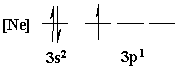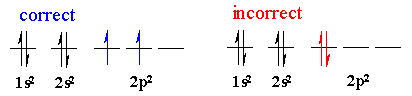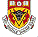Chapter 1: Structure Determines PropertiesOrbitals Quantum numbers
The properties of orbitals (energy, size, shape etc.) and the electrons within the orbitals (location, spin) are described using quantum numbers:

 quantum number symbol value range describes principal n 1, 2, 3, .... energy level angular momentum l 0 to n-1 orbital shape (s = 0, p = 1, d = 2 etc.) magnetic ml - l to +  l spatial orientation and degeneracy (number of orbitals of that type) spin ms ± 1/2 electron spin

Given an orbital, the quantum numbers that describe that orbital can be determined, or, given a set of quantum numbers one can also determine the type of orbital :

 n l ml orbital 1 0 0 1s 2 0 0 2s 1 -1 2px 0 2py +1 2pz

Electron Configurations

e.g. Consider the electron configuration of Al, which is the 13th atom in the periodic table, so atomic number Z = 13 and therefore is has 13 electrons:

A simple electron configuration lists all the orbitals and their electron occupancy
1s22s22p63s23p1
A short form of the above collapses the appropriate noble gas configuration into the atomic symbol for the noble gas
[Ne]3s23p1
Orbital energy diagrams are usually written with a collapsed core.  They are used to indicate how exactly each individual valence orbital is being filled with electrons.Orbital Filling
Electron configurations can be determined using a periodic table and by considering the following as a guide to how the electrons "fill-up" the orbitals:
Aufbau (Building-Up) Principle.  Electrons are placed into the atomic orbitals from lowest to highest energy.  The order of these orbitals is determined using the Schrodinger equation and can be easily determined by looking at the periodic table or using a simple mnemonic.
1s 2s 2p 3s 3p 4s 3d 4p 5s 4d 5p etc......
Hund's rule of Maximum Multiplicity.  When degenerate orbitals are being filled, single electrons are placed into each degenerate orbital before they are paired with another electron in the same orbital.Pauli Exclusion Principle.  No two electrons in a atom can have the same four quantum numbers . This means that only two electrons are allowed in the same orbital, and then they have opposite spin, +1/2 and -1/2.Important distinction:
Valence Electrons are the electrons which are involved in bonding between atoms.  They possess the highest energy and are referred to as the 'outermost' electrons (keep in mind that does not mean they cannot travel close to the nucleus at anyone time but they will be the electrons which travel furthest away from the nucleus).

Core electrons are electrons that under 'normal' reaction conditions are chemically inert.  They are the electrons of an atom which are located in the completely filled energy levels.© Dr. Ian Hunt, Department of Chemistry, University of Calgary